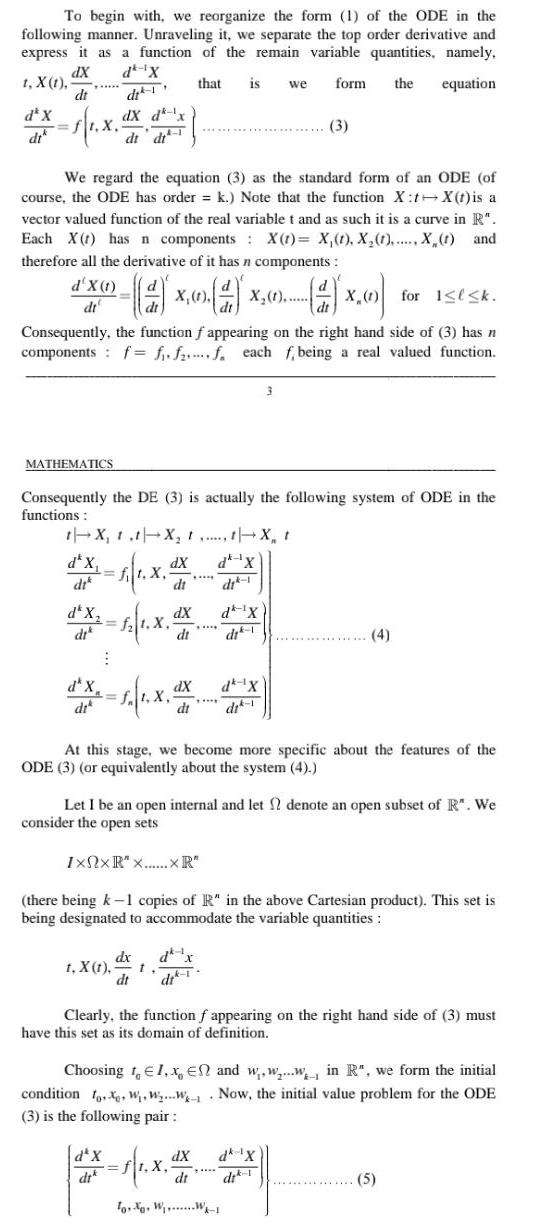Question:

# To begin with we reorganize the form 1 of the ODE in the

Last updated: 9/18/2023To begin with we reorganize the form 1 of the ODE in the following manner Unraveling it we separate the top order derivative and express it as a function of the remain variable quantities namely dX d X t X 1 that is we dt di form the equation ft X dX d x dt di d X di We regard the equation 3 as the standard form of an ODE of course the ODE has order k Note that the function X t X t is a vector valued function of the real variable t and as such it is a curve in R Each X t has n components X t X 1 X 1 X 1 and therefore all the derivative of it has n components d X 1 X 1 X dt X for 1 k Consequently the function f appearing on the right hand side of 3 has n components f f ff each f being a real valued function MATHEMATICS Consequently the DE 3 is actually the following system of ODE in the functions t X t t X X t da X dik 1 ft X d X dik f 1 X d X dik dX dt f t X dx t X t dt 1 d X dik dX dt dx dX dt dit 1 d X di 1 At this stage we become more specific about the features of the ODE 3 or equivalently about the system 4 dit 1 3 3 dk X dik 1 Let I be an open internal and let denote an open subset of R We consider the open sets IxNxR x XR there being k 1 copies of R in the above Cartesian product This set is being designated to accommodate the variable quantities 4 Clearly the function f appearing on the right hand side of 3 must have this set as its domain of definition dX dk X To W W 1 Choosing t 1 x En and www in R we form the initial condition o W WW Now the initial value problem for the ODE 3 is the following pair 5